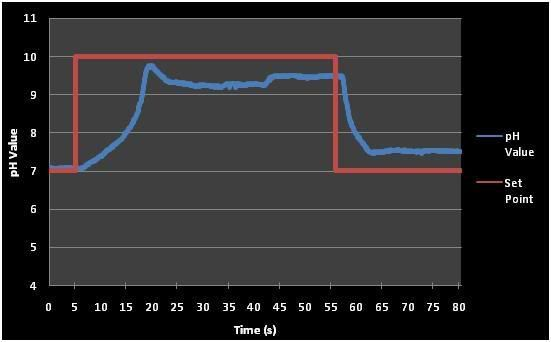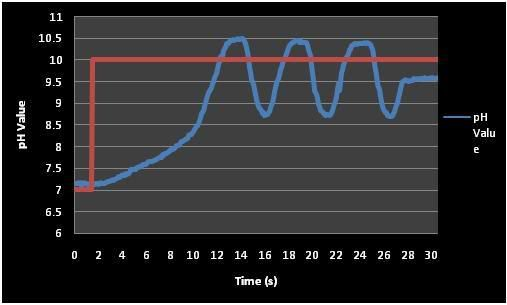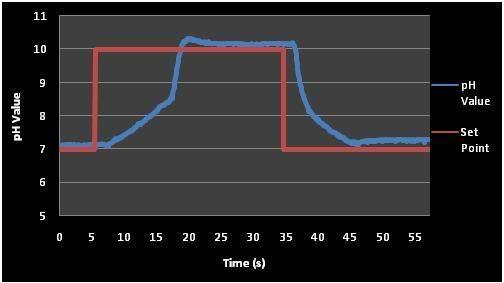Pi Controllert Tuning Results

## PI Controller Tuning Results

In order to obtain good transient response, we need to tune the 2 parameters, K_p and K_i. In our case, we would like to design the control system to be over damped because the time constant is rather large (20min). Since it takes around 20min for the cabbage to change to the desired color, a few seconds on the control system will not make a big difference. Moreover the system makes use of solution which is contained inside the reservoir, so if the system have a high overshoot it will be wasting solution trying to control it back to the setpoint. Using more solution means that the reservoir has to be refilled more of often, so this is not desirable. Below are graphs plotted with different parameters.

## Kp = 4 and Ki = 0.5Figure above shows the graph obtained using Kp = 4 and Ki = 0.5. The output response is not very ideal because of the large steady state error. Hence we move and try to increase the proportional term and see what we get.

## Kp = 14 and Ki = 0.5Figure above shows the graph obtained using Kp = 14 and Ki = 0.5. The output response fluctuates a lot and there is a very high overshoot of 0.5 pH.

## Kp = 7 and Ki = 0.1Figure A\above shows the graph obtained using Kp = 7 and Ki = 0.1. This is by far the best results that we have obtained. There is a very slight overshoot and the error is kept at around 0.1 to 0.2 pH. This is still acceptable because the pH color change over 0.2 pH is not noticeable. Hence this will be parameters we will be using for our control system.

page revision: 1, last edited: 22 Apr 2008 04:41